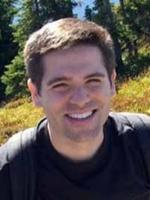# Faculty of MathematicsResearch Interests: Schramm-Loewner evolution, Gaussian free field, Liouville quantum gravity, random planar maps, random walks, mixing times for Markov chains.

## Publications

The geodesics in Liouville quantum gravity are not Schramm–Loewner evolutions
J Miller, W Qian
– Probability Theory and Related Fields
(2020)
177,
677
An almost sure KPZ relation for SLE and Brownian motion
E Gwynne, N Holden, J Miller
– The Annals of Probability
(2020)
48,
527
Conformal invariance of $\CLE_\kappa$ on the Riemann sphere for $\kappa \in (4,8)$
E Gwynne, J Miller, W Qian
– International Mathematics Research Notices
(2020)
Dimension transformation formula for conformal maps into the complement of an SLE curve
E Gwynne, N Holden, J Miller
– Probability Theory and Related Fields
(2020)
176,
649
Non-simple $\SLE$ curves are not determined by their range
J Miller, S Sheffield, W Werner
– Journal of the European Mathematical Society
(2020)
22,
669
The Tutte Embedding of the Poisson–Voronoi Tessellation of the Brownian Disk Converges to $\sqrt{8/3}$-Liouville Quantum Gravity
E Gwynne, J Miller, S Sheffield
– Communications in Mathematical Physics
(2020)
374,
735
Gaussian free field light cones and SLE$_\kappa(\rho)$
J Miller, S Sheffield
– The Annals of Probability
(2019)
47,
3606
Liouville quantum gravity spheres as matings of finite-diameter trees
J Miller, S Sheffield
– Annales de l'Institut Henri Poincaré, Probabilités et Statistiques
(2019)
55,
1712
Uniqueness of the welding problem for SLE and Liouville quantum gravity
O McEnteggart, J Miller, W Qian
– Journal of the Institute of Mathematics of Jussieu
(2019)
1
Metric gluing of Brownian and $\sqrt{8/3}$-Liouville quantum gravity surfaces
E Gwynne, J Miller
– The Annals of Probability
(2019)
47,
2303-
• 1 of 5
• >

## Research Groups

Probability
Applied Probability
Mathematical Finance and Operational Research
Statistical Laboratory

D2.02

01223 337951